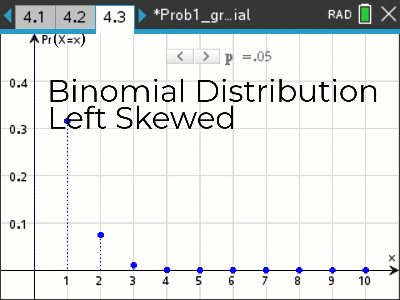# Activities

•• ##### AuthorAust Senior

90 Minutes

• ##### Device
•TI-Nspire™ CX CAS

5.2

## Graphs Binomial Distribution#### Activity Overview

A probability function may be represented as a formula (usually an equation), a table or a graph. In this activity, you will use interactive graphs in TI-Nspire CAS to explore the effect that changing the value of a parameter (a number describing some characteristic of the distribution) has on the graph of that probability function.

#### Objectives

The aim is for students to become familiar with the shape of the binomial distribution for a range of values for n and p, including comparisons of skew and standard deviation and expected value.

#### Vocabulary

• Binomial distribution
• Skew
• Mean
• Standard Deviation